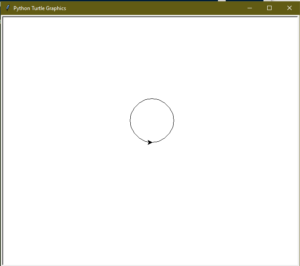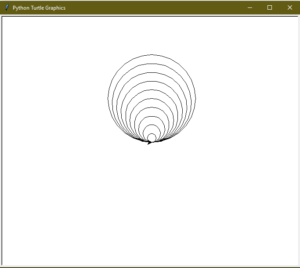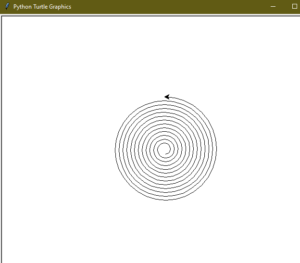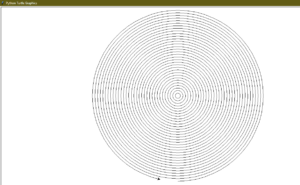Related Articles
Draw Circle in Python using Turtle
• Difficulty Level : Medium
• Last Updated : 16 Jul, 2020

Turtle is a Python feature like a drawing board, which lets us command a turtle to draw all over it! We can use functions like `turtle.forward(…)` and `turtle.right(…)` which can move the turtle around. Turtle is a beginner-friendly way to learn Python by running some basic commands and viewing the turtle do it graphically. It is like a drawing board that allows you to draw over it. The turtle module can be used in both object-oriented and procedure-oriented ways.

To draw, Python `turtle` provides many functions and methods i.e. forward, backward, etc. Some the commonly used methods are:

• forward(x): moves the pen in the forward direction by x unit.
• backward(x): moves the pen in the backward direction by x unit.
• right(x): rotate the pen in the clockwise direction by an angle x.
• left(x): rotate the pen in the anticlockwise direction by an angle x.
• penup(): stop drawing of the turtle pen.
• pendown(): start drawing of the turtle pen.

Now to draw a circle using turtle, we will use a predefined function in “turtle”.

circle(radius): This function draws a circle of the given radius by taking the “turtle” position as the center.

Example:

 `# Python program to demonstrate``# circle drawing`` ` ` ` `import` `turtle``   ` `# Initializing the turtle``t ``=` `turtle.Turtle()`` ` ` ` `r ``=` `50``t.circle(r)`

Output :#### Tangent Circles

A tangent is a line that touches the circumference of a circle from outside at a point, provided that any extension of the line will not cause intersection with the circle. Now, think about a group of circles, that have a common tangent. The group of circles, having common tangent, are known as tangent circles.

Example:

 `# Python program to demonstrate``# tangent circle drawing`` ` ` ` `import` `turtle``   ` `t ``=` `turtle.Turtle()`` ` `# radius for smallest circle``r ``=` `10`` ` `# number of circles``n ``=` `10`` ` `# loop for printing tangent circles``for` `i ``in` `range``(``1``, n ``+` `1``, ``1``):``    ``t.circle(r ``*` `i)`

Output :#### Spiral Circle

Spiral is a shape similar to a circle, except that the radius of the spiral gradually increases after every completed round.

Exmaple:

 `# Python program to demonstrate``# spiral circle drawing`` ` ` ` `import` `turtle``   ` `t ``=` `turtle.Turtle()`` ` `# taking radius of initial radius``r ``=` `10`` ` `# Loop for printing spiral circle``for` `i ``in` `range``(``100``):``    ``t.circle(r ``+` `i, ``45``)`

Output :#### Cocentric Circles

The term concentric is used for a group of things having common. Now Circles having the same center are termed as Concentric Circle.

 `# Python program to demonstrate``# concentric circle drawing`` ` ` ` `import` `turtle``   ` `     ` `t ``=` `turtle.Turtle()`` ` `# radius of the circle``r ``=` `10`` ` `# Loop for printing concentric circles``for` `i ``in` `range``(``50``):``    ``t.circle(r ``*` `i)``    ``t.up()``    ``t.sety((r ``*` `i)``*``(``-``1``))``    ``t.down()`

Output :Attention geek! Strengthen your foundations with the Python Programming Foundation Course and learn the basics.

To begin with, your interview preparations Enhance your Data Structures concepts with the Python DS Course.

My Personal Notes arrow_drop_up